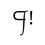# An Exponent Theorem

Let us consider the statement $$b^n - b^{n-1} = b^{n-1} (b-1)$$.

By quotient property of exponents,

$b^n \div b^{n-1} = b$

Subtracting 1 from both sides,

$(b^n \div b^{n-1}) - 1 = b - 1$

$(b^n \div b^{n-1}) - (b^{n-1} \div b^{n-1}) = b - 1$

$(b^n - b^{n-1}) \div b^{n-1} = b - 1$

Multiplying both sides by $b^{n-1}$,

$\boxed{b^n - b^{n-1} = b^{n-1} (b-1)}$

Let $b = \big\{x | x \in \mathbb{R}\big\}$.

If $b = 0$, let $n - 1 = \big\{x | x \in (1,+\infty)\big\}$.4 years, 11 months ago

This discussion board is a place to discuss our Daily Challenges and the math and science related to those challenges. Explanations are more than just a solution — they should explain the steps and thinking strategies that you used to obtain the solution. Comments should further the discussion of math and science.

When posting on Brilliant:

• Use the emojis to react to an explanation, whether you're congratulating a job well done , or just really confused .
• Ask specific questions about the challenge or the steps in somebody's explanation. Well-posed questions can add a lot to the discussion, but posting "I don't understand!" doesn't help anyone.
• Try to contribute something new to the discussion, whether it is an extension, generalization or other idea related to the challenge.

MarkdownAppears as
*italics* or _italics_ italics
**bold** or __bold__ bold
- bulleted- list
• bulleted
• list
1. numbered2. list
1. numbered
2. list
Note: you must add a full line of space before and after lists for them to show up correctly
paragraph 1paragraph 2

paragraph 1

paragraph 2

[example link](https://brilliant.org)example link
> This is a quote
This is a quote
    # I indented these lines
# 4 spaces, and now they show
# up as a code block.

print "hello world"
# I indented these lines
# 4 spaces, and now they show
# up as a code block.

print "hello world"
MathAppears as
Remember to wrap math in $$ ... $$ or $ ... $ to ensure proper formatting.
2 \times 3 $2 \times 3$
2^{34} $2^{34}$
a_{i-1} $a_{i-1}$
\frac{2}{3} $\frac{2}{3}$
\sqrt{2} $\sqrt{2}$
\sum_{i=1}^3 $\sum_{i=1}^3$
\sin \theta $\sin \theta$
\boxed{123} $\boxed{123}$

Sort by:

You can use the property of the exponents. Provided that $b \neq 0$, $b^n-b^{n-1} = b^{n-1}(\frac{b^n}{b^{n-1}}-\frac{b^{n-1}}{b^{n-1}})$ $b^n-b^{n-1} = b^{n-1}(b^{n-(n-1)}-b^{(n-1)-(n-1)})$ $b^n-b^{n-1} = b^{n-1}(b^1-b^0) = b^{n-1}(b-1)$ $Q.E.D.$

- 4 years, 10 months ago

Thank you very much, I have now provided more supportive evidence for my theorem.

- 4 years, 9 months ago

Kay's comment isn't just "supportive evidence". It is a proof of the statement which you stated.

Staff - 4 years, 9 months ago

Thank you, I understand. At first, I was doubtful in a way that others might correct me for calling it "proof" so I decided to call it "supportive evidence."

- 4 years, 9 months ago

Thanks for updating the note!

It can take a while for you to become familiar with mathematical rigor. We all have to start somewhere, and I'm glad that you're learning!

Staff - 4 years, 9 months ago

Note that you have not proven the theorem. You have only demonstrated that it is true in 5 specific cases.

Staff - 4 years, 11 months ago

Thank you for commenting your thoughts on this. This is my first note on Brilliant. Thank you again.

- 4 years, 11 months ago